# NCERT Solutions for Class 7 Maths Chapter 15 Visualising Solid Shapes Ex 15.2

NCERT Solutions for Class 7 Maths Chapter 15 Visualising Solid Shapes Ex 15.2

### NCERT Solutions for Class 7 Maths Chapter 15 Visualising Solid Shapes Ex 15.2

NCERT Solutions for Class 7 Maths Chapter 15 Visualising Solid Shapes Exercise 15.2

Ex 15.2 Class 7 Maths Question 1.
Use isometric dot paper and make an isometric sketch for each one of the given shapes: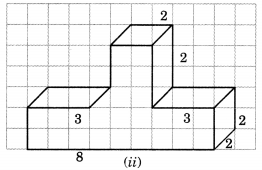Solution: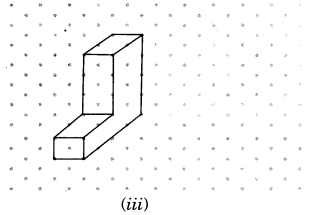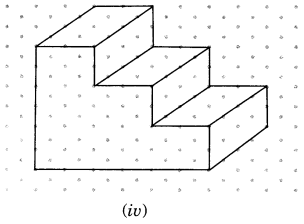Ex 15.2 Class 7 Maths Question 2.
The dimensions of a cuboid are 5 cm, 3 cm and 2 cm. Draw three different isometric sketches of this cuboid.
Solution: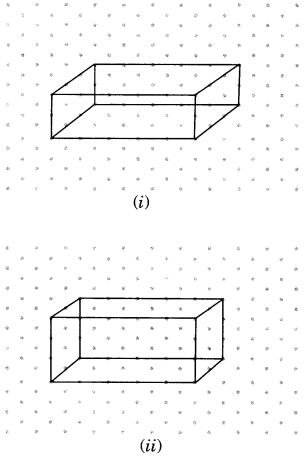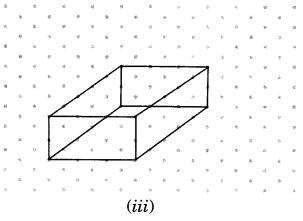Ex 15.2 Class 7 Maths Question 3.
Three cubes with 2 cm edge are placed side by side to form a cuboid. Sketch an oblique or isometric sketch of this cuboid.
Solution:
In the given figure, we have an isometric sketch of a cuboid formed by placing three cubes each of 2 cm edge side by side.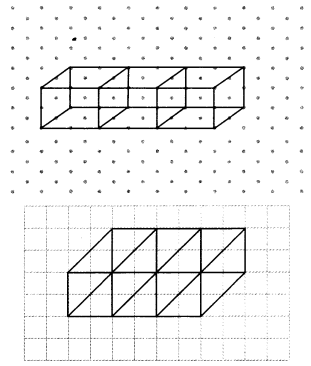Ex 15.2 Class 7 Maths Question 4.
Make an oblique sketch for each one of the given isometric shapes: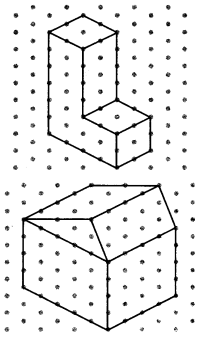Solution: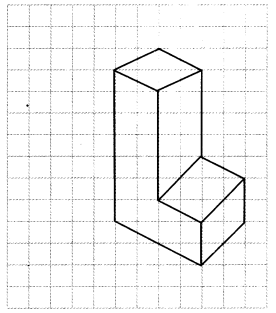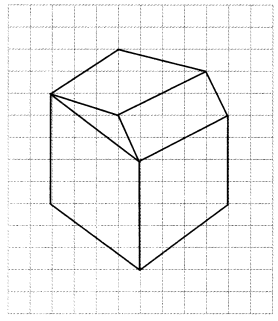Ex 15.2 Class 7 Maths Question 5.
Give (i) an oblique sketch and (ii) an isometric sketch for each of the following:
(а) A cuboid of dimensions 5 cm, 3 cm and 2 cm. (Is your sketch unique?)
(b) A cube with an edge 4 cm long.
An isometric sheet is attached at the end of the book. You could try to make on it some cubes or cuboids of dimensions specified by your friend.
Solution: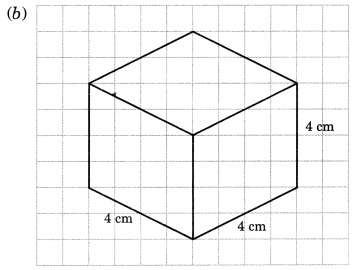No, this sketch is not unique.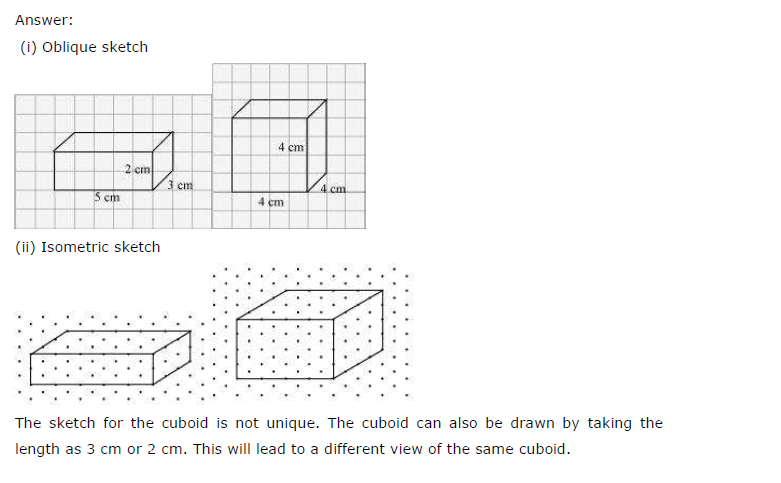## SabDekho

The Complete Educational Website